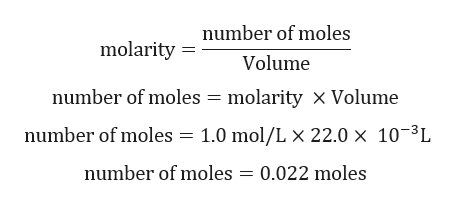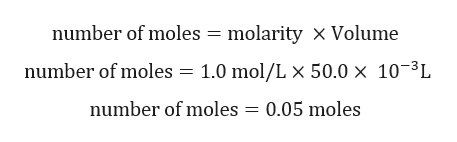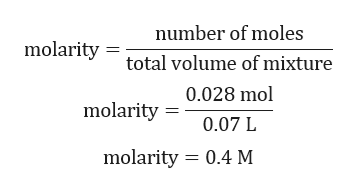# Ka for weak acid HZ is 2.8 * 10^-5. The PH of a buffer prepared by combining 50.0 ml of 1.00 M NaZ and 22.0 ml 1.00 M HZ isA)4.19B) 4.55C) 4.91D) 5.14

Question
30 views

Ka for weak acid HZ is 2.8 * 10^-5. The PH of a buffer prepared by combining 50.0 ml of 1.00 M NaZ and 22.0 ml 1.00 M HZ is

A)4.19

B) 4.55

C) 4.91

D) 5.14

check_circle

Step 1

Calculation for number of moles of HZ:help_outlineImage Transcriptionclosenumber of moles molarity = Volume number of moles = molarity x Volume number of moles = 1.0 mol/L × 22.0 × 10-³L number of moles = 0.022 moles fullscreen
Step 2

Calculation for number of moles of NaZ:help_outlineImage Transcriptionclosenumber of moles = molarity × Volume number of moles = 1.0 mol/L × 50.0 × 10-³L number of moles = 0.05 moles fullscreen
Step 3

Calculation concentration of HZ left after mixing with NaZ:

Number...help_outlineImage Transcriptionclosenumber of moles molarity = total volume of mixture 0.028 mol molarity = 0.07 L molarity = 0.4 M fullscreen

### Want to see the full answer?

See Solution

#### Want to see this answer and more?

Solutions are written by subject experts who are available 24/7. Questions are typically answered within 1 hour.*

See Solution
*Response times may vary by subject and question.
Tagged in

### Equilibrium Concepts